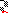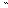# MINLPLib

### A Library of Mixed-Integer and Continuous Nonlinear Programming Instances

#### Instance tln2

 Formatsⓘ ams gms lp mod nl osil pip py Primal Bounds (infeas ≤ 1e-08)ⓘ 5.30000000 p1 ( gdx sol ) (infeas: 0) Other points (infeas > 1e-08)ⓘ Dual Boundsⓘ 5.30000000 (ANTIGONE)5.30000000 (BARON)5.30000000 (COUENNE)5.30000000 (GUROBI)5.30000000 (LINDO)5.30000000 (SCIP)5.30000000 (SHOT) Referencesⓘ Harjunkoski, Iiro, Westerlund, Tapio, Pörn, Ray, and Skrifvars, Hans, Different Transformations for Solving Non-Convex Trim Loss Problems by MINLP, European Journal of Operational Research, 105:3, 1998, 594-603. Sourceⓘ MacMINLP model trimlon.mod with trimlon2.dat Applicationⓘ Trim loss minimization problem Added to libraryⓘ 01 May 2001 Problem typeⓘ MIQCP #Variablesⓘ 8 #Binary Variablesⓘ 2 #Integer Variablesⓘ 6 #Nonlinear Variablesⓘ 6 #Nonlinear Binary Variablesⓘ 0 #Nonlinear Integer Variablesⓘ 6 Objective Senseⓘ min Objective typeⓘ linear Objective curvatureⓘ linear #Nonzeros in Objectiveⓘ 4 #Nonlinear Nonzeros in Objectiveⓘ 0 #Constraintsⓘ 12 #Linear Constraintsⓘ 10 #Quadratic Constraintsⓘ 2 #Polynomial Constraintsⓘ 0 #Signomial Constraintsⓘ 0 #General Nonlinear Constraintsⓘ 0 Operands in Gen. Nonlin. Functionsⓘ Constraints curvatureⓘ indefinite #Nonzeros in Jacobianⓘ 28 #Nonlinear Nonzeros in Jacobianⓘ 8 #Nonzeros in (Upper-Left) Hessian of Lagrangianⓘ 8 #Nonzeros in Diagonal of Hessian of Lagrangianⓘ 0 #Blocks in Hessian of Lagrangianⓘ 2 Minimal blocksize in Hessian of Lagrangianⓘ 3 Maximal blocksize in Hessian of Lagrangianⓘ 3 Average blocksize in Hessian of Lagrangianⓘ 3.0 #Semicontinuitiesⓘ 0 #Nonlinear Semicontinuitiesⓘ 0 #SOS type 1ⓘ 0 #SOS type 2ⓘ 0 Minimal coefficientⓘ 1.0000e-01 Maximal coefficientⓘ 5.7000e+02 Infeasibility of initial pointⓘ 670 Sparsity JacobianⓘSparsity Hessian of Lagrangianⓘ```\$offlisting
*
*  Equation counts
*      Total        E        G        L        N        X        C        B
*         13        1        0       12        0        0        0        0
*
*  Variable counts
*                   x        b        i      s1s      s2s       sc       si
*      Total     cont   binary  integer     sos1     sos2    scont     sint
*          9        1        2        6        0        0        0        0
*  FX      0
*
*  Nonzero counts
*      Total    const       NL      DLL
*         33       25        8        0
*
*  Solve m using MINLP minimizing objvar;

Variables  b1,b2,i3,i4,i5,i6,i7,i8,objvar;

Binary Variables  b1,b2;

Integer Variables  i3,i4,i5,i6,i7,i8;

Equations  e1,e2,e3,e4,e5,e6,e7,e8,e9,e10,e11,e12,e13;

e1..  - 0.1*b1 - 0.2*b2 - i3 - i4 + objvar =E= 0;

e2..    460*i5 + 570*i7 =L= 1900;

e3..    460*i6 + 570*i8 =L= 1900;

e4..  - 460*i5 - 570*i7 =L= -1700;

e5..  - 460*i6 - 570*i8 =L= -1700;

e6..    i5 + i7 =L= 5;

e7..    i6 + i8 =L= 5;

e8..    b1 - i3 =L= 0;

e9..    b2 - i4 =L= 0;

e10..  - 15*b1 + i3 =L= 0;

e11..  - 15*b2 + i4 =L= 0;

e12.. -(i3*i5 + i4*i6) =L= -8;

e13.. -(i3*i7 + i4*i8) =L= -7;

* set non-default bounds
i3.up = 15;
i4.up = 15;
i5.up = 5;
i6.up = 5;
i7.up = 5;
i8.up = 5;

* set non-default levels
i3.l = 1;
i4.l = 1;
i5.l = 1;
i6.l = 1;
i7.l = 1;
i8.l = 1;

Model m / all /;

m.limrow=0; m.limcol=0;
m.tolproj=0.0;

\$if NOT '%gams.u1%' == '' \$include '%gams.u1%'

\$if not set MINLP \$set MINLP MINLP
Solve m using %MINLP% minimizing objvar;

```

Last updated: 2023-06-01 Git hash: fdbb0df7